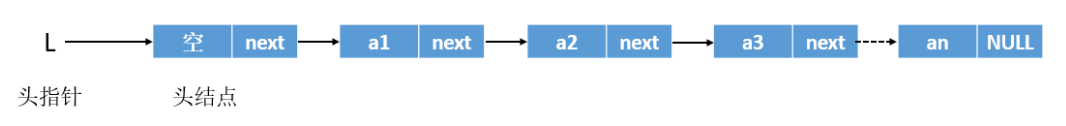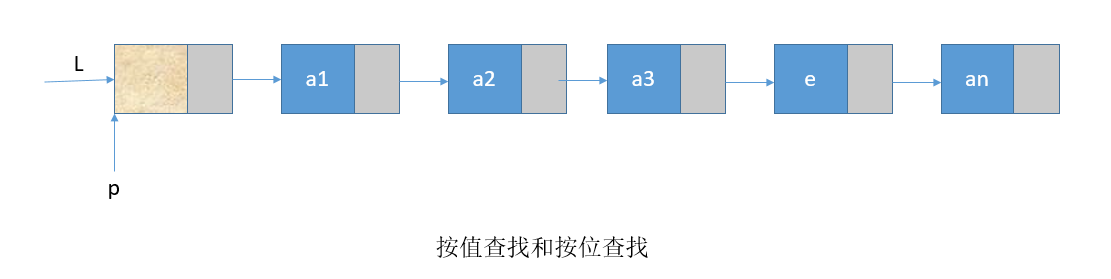V1

2023/02/05阅读：26主题：萌绿

# 【数据结构】线性表的链式表示—单链表

C语言合集

### 1. 单链表的定义

``typedef struct LNode{ // 单链表结点类型    ElemType data;  // 数据域    struct LNode *next;  // 指针域}LNode,*LinkList;``

• typedef相当于起别名，具体用法参考C语言的文章。
• LNode强调这是一个结点。*LinList强调这是一个单链表。头指针：链表中第一个节点的存储位置，用来标识单链表。

### 3. 单链表的基本操作

#### 插入操作

• 创建新结点
``q=(LNode*)malloc(sizeof(LNode))q->data=x;``
• 头插入和中间插入
``q->next=p->next;p->next=q;``
• 表尾插入
``p->next=q;q->next=NULL;``

#### 删除操作

``p=GetElem(L,i-1); // 查找删除位置的前驱结点p->next=q->next;free(q);``

#### 按位查找

``LNode *p=L->next;int j=1;while(p && j<i){    p=p->next;    j++;}return p;``

#### 按值查找

``LNode *p=L->next;while(p!=NULL && p->data!=e){    p=p->next;}return p;``说明：L表示头指针，用来指向头结点。

V1